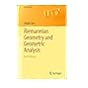Normal view

Riemannian Geometry and Geometric Analysis [electronic resource] / by Jürgen Jost.

Material type:TextSeries: Universitext: Publisher: Berlin, Heidelberg : Springer Berlin Heidelberg, 2011Description: XIII, 611 p. 16 illus., 4 illus. in color. online resourceContent type: text Media type: computer Carrier type: online resourceISBN: 9783642212987Additional physical formats: Printed edition:: No titleDDC classification: 516.36 LOC classification: QA641-670Online resources: Click here to access online
Contents:
1. Riemannian Manifolds -- 2. Lie Groups and Vector Bundles -- 3. The Laplace Operator and Harmonic Differential Forms -- 4. Connections and Curvature -- 5. Geodesics and Jacobi Fields -- 6. Symmetric Spaces and K¨ahler Manifolds -- 7. Morse Theory and Floer Homology -- 8. Harmonic Maps between Riemannian Manifolds -- 9. Harmonic Maps from Riemann Surfaces -- 10. Variational Problems from Quantum Field Theory -- A. Linear Elliptic Partial Differential Equations -- A.1 Sobolev Spaces -- A.2 Linear Elliptic Equations -- A.3 Linear Parabolic Equations -- B. Fundamental Groups and Covering Spaces -- Bibliography -- Index.
Summary: This established reference work continues to lead its readers to some of the hottest topics of contemporary mathematical research. The previous edition already introduced and explained the ideas of the parabolic methods that had found a spectacular success in the work of Perelman at the examples of closed geodesics and harmonic forms. It also discussed further examples of geometric variational problems from quantum field theory, another source of profound new ideas and methods in geometry. The 6th edition includes a systematic treatment of eigenvalues of Riemannian manifolds and several other additions. Also, the entire material has been reorganized in order to improve the coherence of the book. From the reviews: "This book provides a very readable introduction to Riemannian geometry and geometric analysis. ... With the vast development of the mathematical subject of geometric analysis, the present textbook is most welcome." Mathematical Reviews "...the material ... is self-contained. Each chapter ends with a set of exercises. Most of the paragraphs have a section ‘Perspectives’, written with the aim to place the material in a broader context and explain further results and directions." Zentralblatt MATH  .
Tags from this library: No tags from this library for this title.Average rating: 0.0 (0 votes)
Item type Current location Collection Call number Status Date due Barcode Item holdseBook e-Library

Electronic Book@IST

EBook Available
Total holds: 0

1. Riemannian Manifolds -- 2. Lie Groups and Vector Bundles -- 3. The Laplace Operator and Harmonic Differential Forms -- 4. Connections and Curvature -- 5. Geodesics and Jacobi Fields -- 6. Symmetric Spaces and K¨ahler Manifolds -- 7. Morse Theory and Floer Homology -- 8. Harmonic Maps between Riemannian Manifolds -- 9. Harmonic Maps from Riemann Surfaces -- 10. Variational Problems from Quantum Field Theory -- A. Linear Elliptic Partial Differential Equations -- A.1 Sobolev Spaces -- A.2 Linear Elliptic Equations -- A.3 Linear Parabolic Equations -- B. Fundamental Groups and Covering Spaces -- Bibliography -- Index.

This established reference work continues to lead its readers to some of the hottest topics of contemporary mathematical research. The previous edition already introduced and explained the ideas of the parabolic methods that had found a spectacular success in the work of Perelman at the examples of closed geodesics and harmonic forms. It also discussed further examples of geometric variational problems from quantum field theory, another source of profound new ideas and methods in geometry. The 6th edition includes a systematic treatment of eigenvalues of Riemannian manifolds and several other additions. Also, the entire material has been reorganized in order to improve the coherence of the book. From the reviews: "This book provides a very readable introduction to Riemannian geometry and geometric analysis. ... With the vast development of the mathematical subject of geometric analysis, the present textbook is most welcome." Mathematical Reviews "...the material ... is self-contained. Each chapter ends with a set of exercises. Most of the paragraphs have a section ‘Perspectives’, written with the aim to place the material in a broader context and explain further results and directions." Zentralblatt MATH  .

There are no comments for this item.Riemannian Geometry and Geometric Analysis (Universitext). by Jost, Jürgen. ©2011